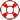Standard:

Description:

Standard:

# Math.2.NBT.7 or 2.NBT.B.7

Description:

## Add and subtract within 1000, using concrete models or drawings and strategies based on place value, properties of operations, and/or the relationship between addition and subtraction; relate the strategy to a written method. Understand that in adding or subtracting three-digit numbers, one adds or subtracts hundreds and hundreds, tens and tens, ones and ones; and sometimes it is necessary to compose or decompose tens or hundreds.2nd Grade Math - Subtract Within 1000 Lesson